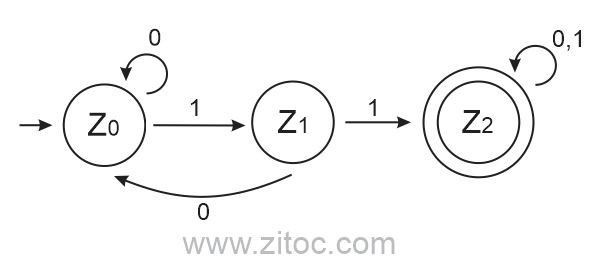# FA to Regular Expressions construction

This topic designed for finite automata FA to regular expression (RE) construction.

Case 1:

Finite Automata for RE = 0

For a regular expression (RE) ‘0’, we can construct the following FA −.Case 2:

Finite Automata for RE = 01

For a RE ‘01’, we can construct the following FA −.Case 3:

Finite Automata for RE = (0+1)

For a RE ‘(0+1)’, we can construct the following −.Case 4:

Finite Automata for RE = (0+1)*

For a RE ‘(0+1)*’, we can construct the following –.Case 5:

Finite Automata for RE = 0(0+1)*1

This expression can also be known as starting from ‘0’ and ending on ‘1’.Case 6:

Finite Automata for RE = 1(0+1)*0

This expression can also be known as starting from ‘1’ and ending on ‘0’.Case 7:

Finite Automata for RE = 1(0+1)*0 + 0(0+1)*1

This expression can also be known as starting and ending on different points.Case 8:

Finite Automata for RE = (0+1)*11(0+1)*

This expression can also be known as a string containing double ‘11’.Case 9:

Finite Automata for “Exactly one 0 and two 11”.Regular Language